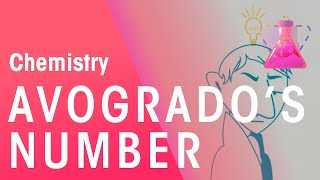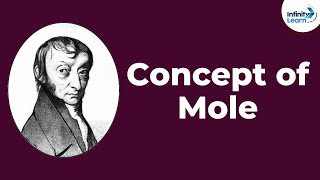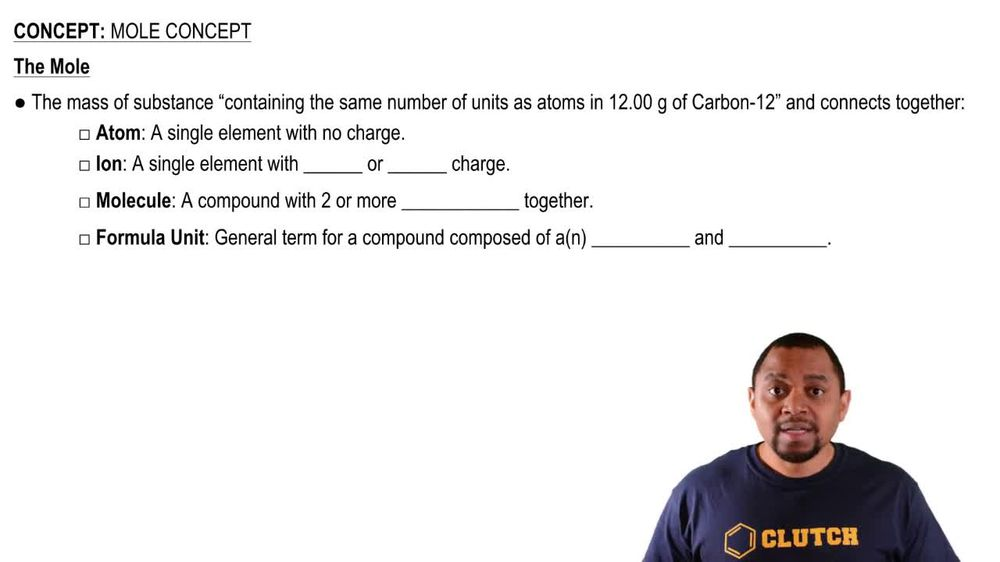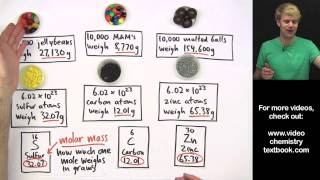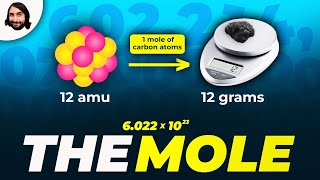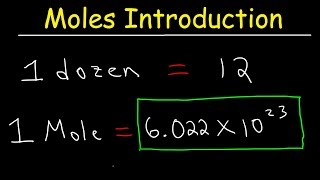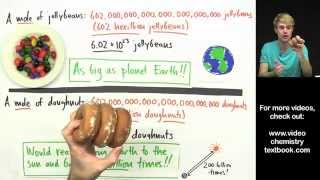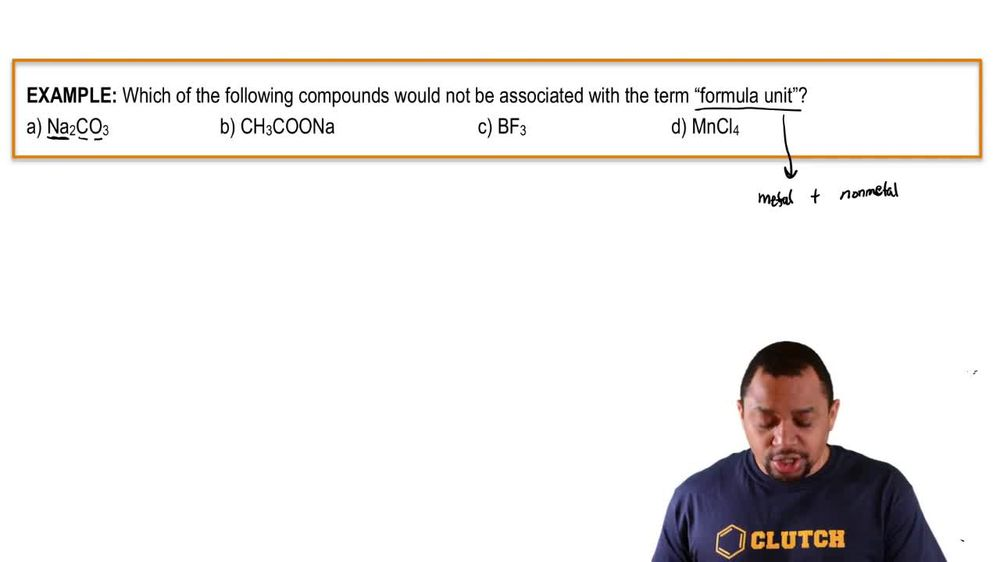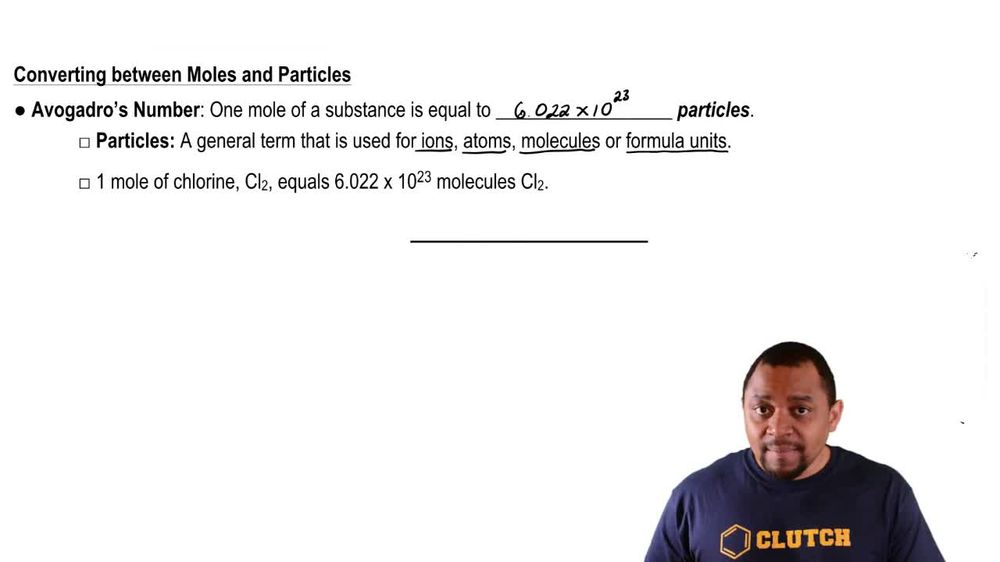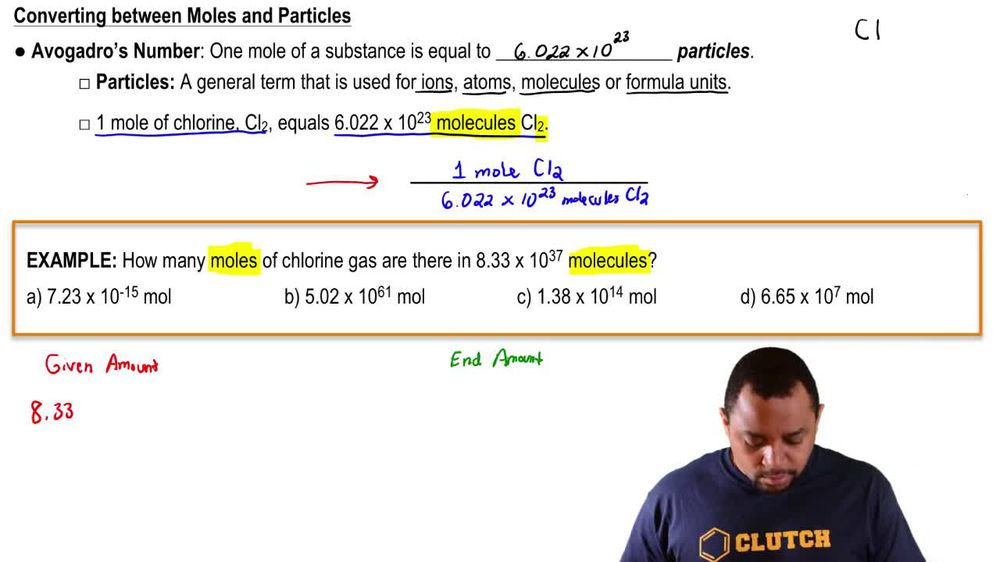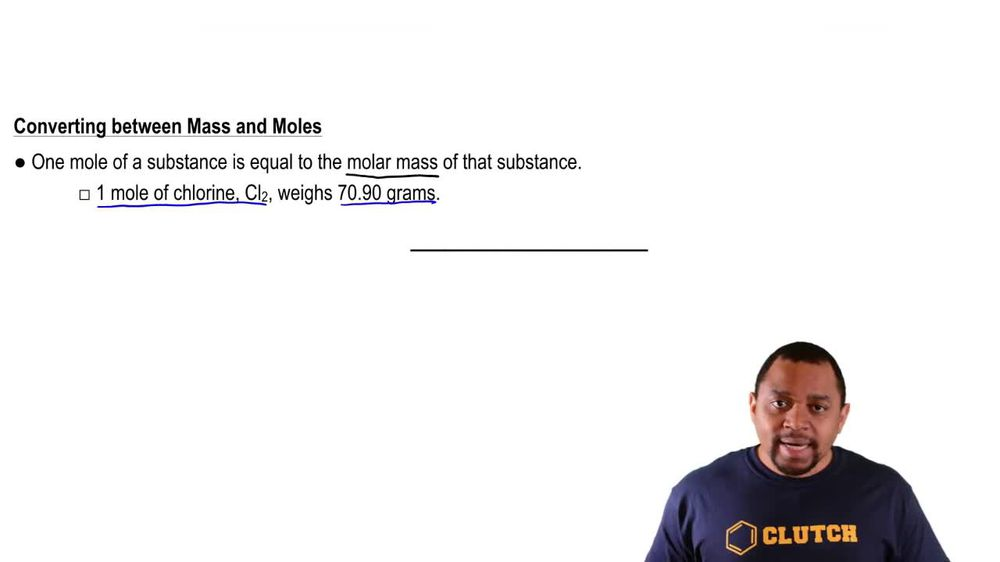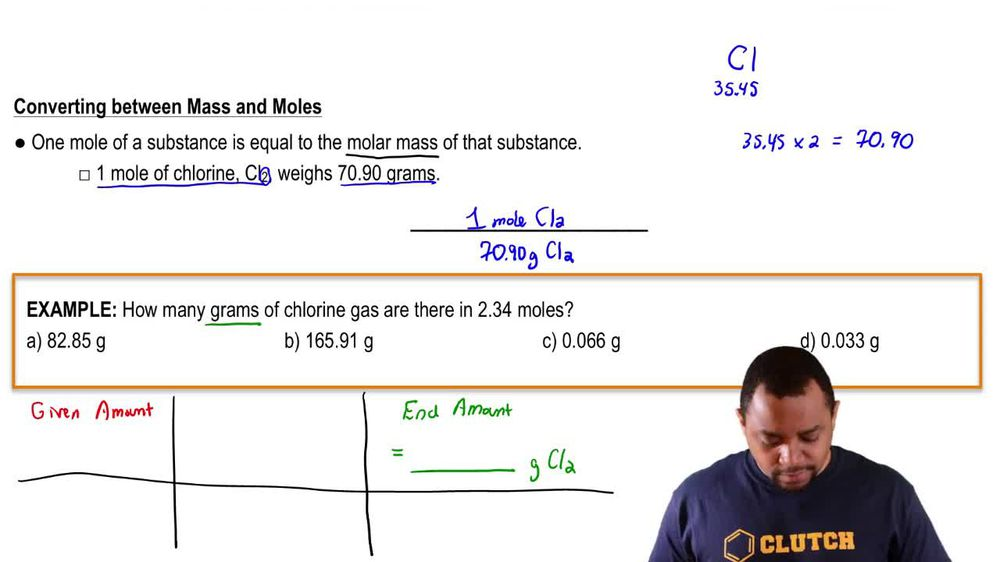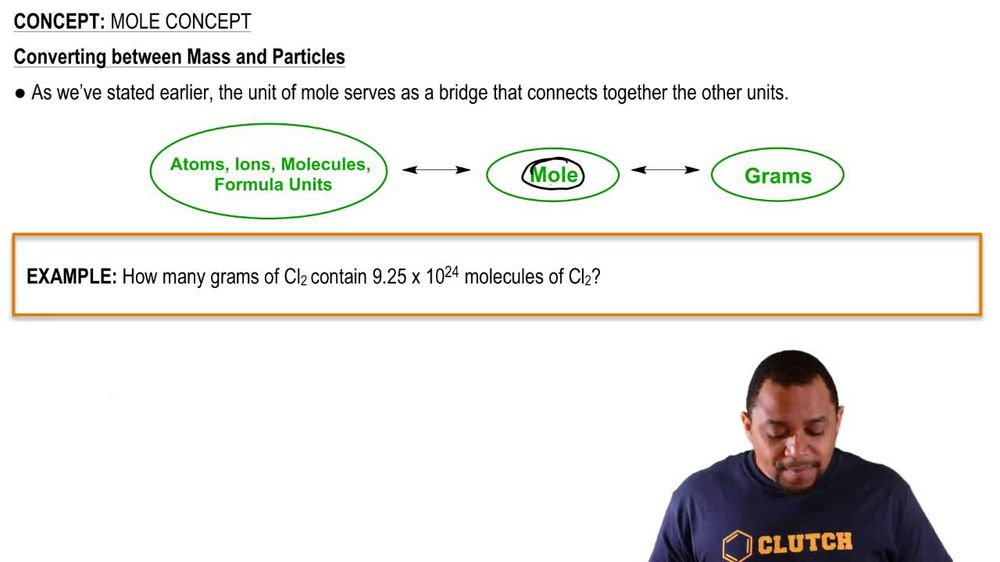Start typing, then use the up and down arrows to select an option from the list.
1. 2. Atoms & Elements2. Mole Concept# Mole Concept

by Jules Bruno
119 views
0
Just as the mall connect is the connection toe particles, we can use moles as the connection to Mass. Now we're going to stay here. One mole of a substance is equal to the molar mass of that substance. Remember the term Mueller, Mass. We can use Moeller Wait. Molecular weight or molecular mass? They all mean the same thing here. We're told that one mole off still chlorine weighs 70.90 g. What does that 70.90 g come from? Well, if you look on the periodic table, you'll see chlorine and you'll see that underneath chlorine or above it, you'll see ah, value of 35.45. That's the mass of one chlorine. But here we're dealing with two chlorine that be 35. times to, which gives us that value of 70.90 now. Here again, this can serve as a conversion factor in itself, where one mole of C L two is 70. g C. L two, and since it's a conversion factor, we can also flip it where 70.90 g of C. L two on top and one mole of CL two on the bottom. Remember, we do this based on if we need to cancel out certain units. So just remember, the moles can serve as a bridge to connect us to the mass off element or compound. Now that we've seen this, let's move on to our example problem.